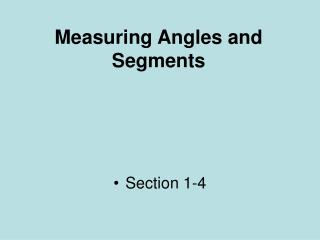DownloadDownload PresentationMeasuring Angles and Segments

# Measuring Angles and Segments

Télécharger la présentation## Measuring Angles and Segments

- - - - - - - - - - - - - - - - - - - - - - - - - - - E N D - - - - - - - - - - - - - - - - - - - - - - - - - - -
##### Presentation Transcript

1. Measuring Angles and Segments • Section 1-4

2. Measuring Segments • The distance between any two points on a horizontal or vertical line • ABSOLUTE VALUE OF THE DIFFERENCE OF THE COORDINATES • AB = |a – b|

3. Congruent Segments • Two or more segments with the same length • Figures are congruent • Measures are equal

4. Postulate 1-6Segment Addition Postulate • If three points A, B, and C are collinear, and B is between A and C, then: AB + BC = AC B A C

5. If DT = 60, find the value of x. Then find DS and St 2x - 8 3x - 12 D S T

6. Angles Right Angle – angle with measure of 90 degrees Acute Angle – angle with measure less than 90 degrees Obtuse Angle – angle with measure greater than 90 degrees but less than180 degrees Straight Angle – angle with measure of 180 degrees

7. Postulate 1-8Angle Addition Postulate • If point B is in the interior of <AOC, then m<AOB + m<BOC = m<AOC B A O C

8. Postulate 1-8**Angle Addition Postulate** • If <AOC is a straight angle, then m<AOB + m<BOC = 180 B O C A

9. Congruent Angles • Angles with the same measure • Figures are congruent • Measures are equal

10. Angles • Degree • Unit of measure for measuring angles • Protractor • Instrument used to measure angles